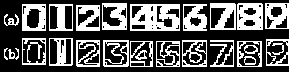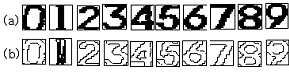## Lncs 3496 - enhanced fuzzy single layer perceptron

Enhanced Fuzzy Single Layer Perceptron
Kwangbaek Kim1, Sungshin Kim2, Younghoon Joo3, and Am-Sok Oh4 1 Dept. of Computer Engineering, Silla University, Korea 2 School of Electrical and Computer Engineering, Pusan National University, Korea 3 School of Electronic and Information Engineering, Kunsan National University, Korea 4 Dept. of Multimedia Engineering, Tongmyong Univ. of Information Technology, Korea Abstract. In this paper, a method of improving the learning time and conver-
gence rate is proposed to exploit the advantages of artificial neural networks
and fuzzy theory to neuron structure. This method is applied to the XOR prob-
lem, n bit parity problem, which is used as the benchmark in neural network
structure, and recognition of digit image in the vehicle plate image for practical
image recognition. As a result of experiments, it does not always guarantee the
convergence. However, the network was improved the learning time and has the
high convergence rate. The proposed network can be extended to an arbitrary
layer. Though a single layer structure is considered, the proposed method has a
capability of high speed during the learning process and rapid processing on
huge patterns.
1 Introduction
In the conventional single layer perceptron, it is inappropriate to use when a decision boundary for classifying input pattern does not composed of hyperplane. Moreover, the conventional single layer perceptron, due to its use of unit function, was highly sensitive to change in the weights, difficult to implement and could not learn from past data . Therefore, it could not find a solution of the exclusive OR problem, the benchmark. There are a lot of endeavor to implement a fuzzy theory to artificial neu-ral network . Goh et al.  proposed the fuzzy single layer perceptron algorithm, and advanced fuzzy perceptron based on the generalized delta rule to solve the XOR problem, and the classical problem. This algorithm guarantees some degree of stabil-ity and convergence in application using fuzzy data, however, it causes an increased amount of computation and some difficulties in application of the complicated image recognition. However, the enhanced fuzzy perceptron has shortcomings such as the possibility of falling in local minima and slow learning time . In this paper, we propose an enhanced fuzzy single layer perceptron. We construct, and train, a new type of fuzzy neural net to model the linear function. Properties of this new type of fuzzy neural net include: (1) proposed linear activation function; and (2) a modified delta rule for learning. We will show that such properties can guarantee to find solutions for the problems-such as exclusive OR, 3 bits parity, 4 bits parity and digit image recognition on which simple perceptron and simple fuzzy perceptron can not. J. Wang, X. Liao, and Z. Yi (Eds.): ISNN 2005, LNCS 3496, pp. 603–608, 2005. Springer-Verlag Berlin Heidelberg 2005 2 A Fuzzy Single Layer Perceptron
A proposed learning algorithm can be simplified and divided into four steps. For each input, repeat step 1, step 2, step 3, and step 4 until error is minimized. Step 1: Initialize weight and bias term. Define, W 1 ≤ ≤ to be the weight from input j to output i at time t, and ) to small random values, thus initializing all the weights and bias term. Step 2: Rearrange i A according to the ascending order of membership degree m j and m at the beginning of this sequence. 0.0 = m m ≤ ! ≤ m m ≤ 1 0 Compute the consecutive difference between the items of the sequence. − , where k = 0,!,n Step 3: Calculate a soma (O 's actual output. θ is linear activation function, where i = ,1!,I In the sigmoid function, if the value of  Therefore, the proposed linear activation function expression is represented as fol-  ∑ ij + i  > 5 0 × ∑ ij + i  + 0. , . ≤  ∑ ij + i  ≤ 5. , Enhanced Fuzzy Single Layer Perceptron 605 The formulation of the activation linear function is follow. where the range means monotonic increasing internal except for the interval between 0.0 and 1.0 of value of the  J −1 Step 4: Applying the modified delta rule. And we derive the incremental changes for weight and bias term. = i × i × ∑ k × ∑ ij i i × ∆Wij (t) 1 = ηi × Ei × f i )+αi × ∆θi (t) 1 = θi (t)+ ∆θi (t + ) Finally, we enhance the training speed by using the dynamical learning rate and Inactivati totalsoma Activationtotalsoma > 0) 1 = i(t)+ ∆ i(t + ) Inactivati totalsoma Activationtotalsoma > 0) 1 = i(t)+ ∆ i(t + ) 2.1 Error Criteria Problem by Division of Soma
In the conventional learning method, learning is continued until squared sum of error is smaller than error criteria. However, this method is contradictory to the physiologi-cal neuron structure and takes place the occasion which a certain soma's output is not any longer decreased and learn no more . The error criteria was divided into activa-tion and inactivation criteria. One is an activation soma's criterion of output “1”, the other is an inactivation soma's of output “0”. The activation criterion is decided by soma of value “1” in the discriminate problem of actual output patterns, which means in physiological analysis that the pattern is classified by the activated soma. In this case, the network must be activated by activated somas. The criterion of activated soma can be set to the range of [0,0.1]. In this paper, however, the error criterion of activated soma was established as 0.05. On the other hand, the error criterion of inactivation soma was defined as the squared error sum, the difference between output and target value of the soma. Fig.1 shows the proposed algorithm. 3 Simulation and Result
We simulated the proposed method on IBM PC/586 with VC++ language. In order to evaluate the proposed algorithm, we applied it to the exclusive OR, 3 bits parity while ((Activation_no == Target_activated_no) &&(Inactivation_error <= Inactivation_area)) do { for (i=0; i<Pattern_no; i++) for(j=0; j<Out_cell_no;j++) { Forward Pass; Backward Pass; if(Out_cell=Activation_soma&&|error|<= Activation_area) Activation_number++; if (Out_cell=Inactivation_soma) Inactivation_error += error * error; } } Fig. 1. Learning algorithm by division of soma.
which is a benchmark in neural network and pattern recognition problems, a kind of image recognition. In the proposed algorithm, the error criterion of activation and inactivation for soma was set to 0.09. 3.1 Exclusive OR and 3 Bits Parity
Here, we set up initial learning rate and initial momentum as 0.5 and 0.75, respec-tively. Also we set up the range of weight [0,1]. In general, the range of weights were [-0.5,0.5] or [-1,1]. As shown in Table 1 and Table 2, the proposed method showed higher performance than the conventional fuzzy single layer perceptron in conver-gence epochs and convergence rates of the three tasks. Table 1. Convergence rate in initial weight range.
Table 2. Comparison of step number.
3.2 Digit Image Recognition
We extracted digit images in a vehicle plate using the method in . We extracted the license plate from the image using the characteristic that green color area of the li-cense plate is denser than other colors. The procedure of image pre-processing is presented in Fig.2 and the example images we used are shown in Fig.3.Enhanced Fuzzy Single Layer Perceptron 607 Image
BMP
Proposed Smoothing
Proposed
Recognition
Pattern
File
& Edge Detection
Learning Algorithm
Fig. 2. Preprocessing diagram.
Fig. 3. (a) Digit image and (b) Training image by edge detection.
We carried out image pre-processing in order to prevent high computational load as well as loss of information. If the extracted digit images were used as training pat-terns, it requires expensive computation load. In contrast skeleton method causes loss of important information of images. To overcome this trade-off, we used edge infor-mation of images. The most-frequent value method was used for image pre-processing. This method was used because blurring of boundary using common smoothing methods. Thus, it degrades both color and contour lines. The new method replaced a pixel's value with the most frequent value among specific neighboring pixels. If the difference of abso-lute value between neighborhoods is zero in a given area, the area was considered as background. Otherwise, it was considered as a contour. This contour was used as a training pattern. The input units were composed of 32 × 32 array for image patterns. In simulation, the fuzzy perceptron was not converged, but the proposed method was converged on 70 steps at image patterns. Table 3 is shown the summary of the results in training epochs between two algorithms. Table 3. The comparison of epoch number.
4 Conclusions
The study and application of fusion fuzzy theory with logic and inference and neural network with learning ability have been actually achieving according to expansion of automatic system and information processing, etc. We have proposed a fuzzy supervised learning algorithm which has greater stabil- ity and functional varieties compared with the conventional fuzzy single layer percep-tron. The proposed network is able to extend the arbitrary layers and has high conver-gence in case of two layers or more. Though, we considered only the case of single layer, the networks had the capability of high speed during the learning process and rapid processing on huge patterns. The proposed algorithm shows the possibility of the application to the image recognition besides benchmark test in neural network by single layer structure. In future study, we will develop a novel fuzzy learning algorithm and apply it to References
1. Rosenblatt, F.: The Perceptron: A Perceiving and Recognizing Automaton. Cornell Univ. Ithaca. NY. Project PARA Cornell Aeronaut Lab. Rep (1997) 85-460 2. Gupta, M. M. and Qi, J.: On Fuzzy Neuron Models. Proceedings of IJCNN. 2 (1991) 431-435
3. Goh, T. H., Wang, P. Z., and Lui, H. C.: Learning Algorithm for Enhanced Fuzzy Perceptron.
Proceedings of IJCNN, 2 (1992) 435-440
4. Kim, K. B. and Cha, E. Y.: A New Single Layer Perceptron Using Fuzzy Neural Control- ler. Simulators International XII, 27 (1995) 341-343
5. Kim, K. B., Joo, Y. H., and Cho, J. H.: An Enhanced Neural Network. Lecture Notes in Computer Science, LNCS 3320 (2004) 176-179
6. Kim, K, B., Kim, M. H., and Lho, Y. U.: Character Extraction of Car License Plates Using RGB Color Information and Fuzzy Binarization. Journal of The Korean Institute of Mari-
time Information & Communication Sciences, 8 (2004) 80-87

Source: http://raic.kunsan.ac.kr/paper/Ij/IJ-2005-33.pdf

### How i treat mpns.

How I treat MPNs. Commentary on the season's scientif ic f indings and MPN events. In this concluding section of diagnosis and treatment of MPNs, four of the world’s pre-eminent MPN researchers and clinical specialists combine current thinking from Europe and the United States. Dr. Claire Harrison, Dr. Hans Hasselbalch, Dr. Richard Silver and Dr. Ruben Mesa share their approach to trea

### Microsoft word - southern sandstone supervisors award technical notes

Southern Sandstone Supervisors Award - Syllabus and Technical Notes 1. Knowledge a) Keep up to date with current practices eg reading magazines, talking to climbers/group leaders. b) Have an understanding of the various sub-sports within climbing. c) Be aware of the need for warming up and how to avoid injuries. d) Be familiar with the equipment available. 2. Personal Climbing a